PHP error in Ad Inserter block 1 - Block 1

# CBSE Sample Papers for Class 12 Maths Solved 2016 Set 2

0
1072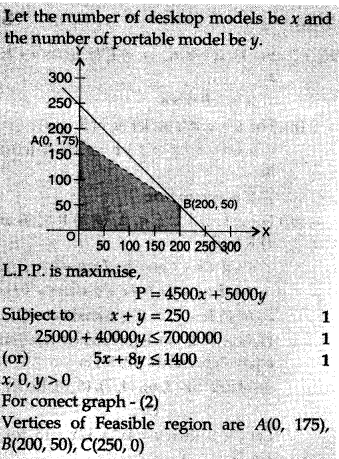SECTION-A

Question numbers 1 to 6 carry 1 mark each.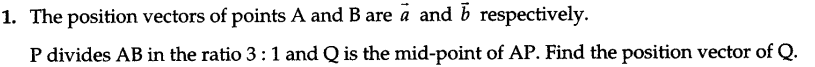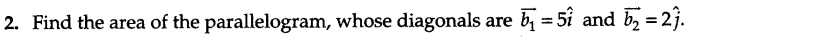3.If P(2, 3, 4) is the foot the of the perpendicular from the origin to a plane, then write the vector equation of this plane.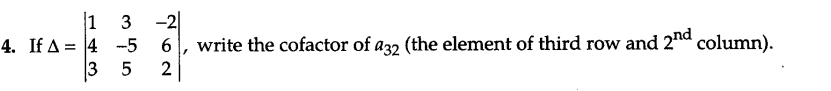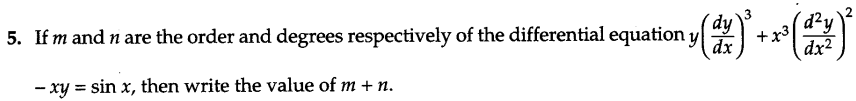6.Write the differential equation representing the curve y2 = 4ax, where a is an arbitrary constant.

SECTION – B

Question numbers 7 to 19 carry 4 marks each.
7.To raise money for an orphanage, students of three schools A, B and C organized an exhibition in their locality, where they sold paper bags, scrap-books and pastel sheets made by them using recycled paper, at the rate of Rs 20, Rs 15 and Rs 5 per unit respectively. School A sold 25 paper-bags, 12 scrap-books and 34 pastel sheets. School B sold 22 paper-bags, 15 scrapbooks and 28 pastel-sheets while school C sold 26 paper-bags, 18 scrap-books and 36 pastel sheets. Using matrices, find the total amount raised by each school.By such exhibition, which values are inculcated in the students ?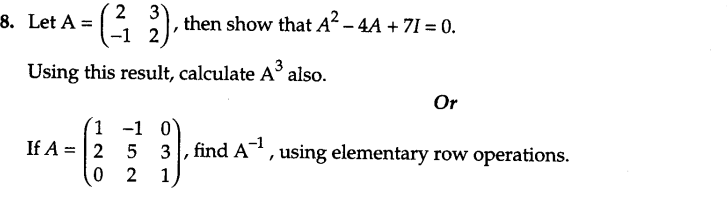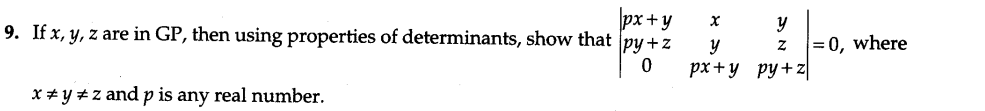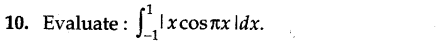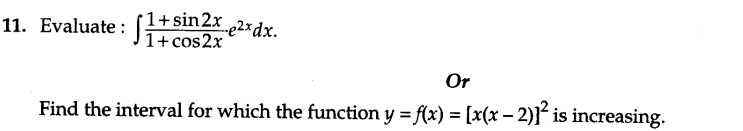12.Consider the experiment of tossing a coin. If the coin shows tail, toss it again but if it shows head, then throw a die. Find the conditional probability of the event that ‘the die shows a number greater than 3’ given that ‘there is at least one head’.
Or
How many times must a man toss a fair coin so that the probability of having at least one head is more than 90% ?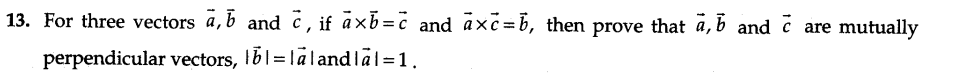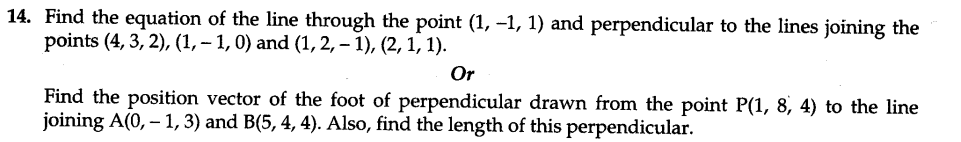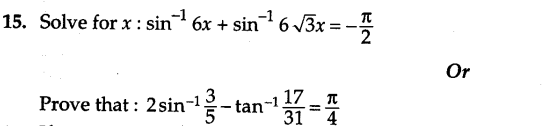16.If x = sin t, y = sin kt, show that,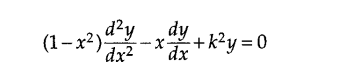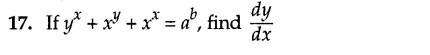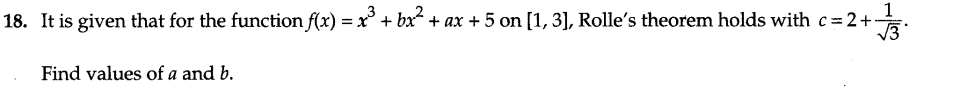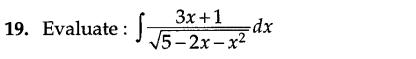SECTION – C

Question numbers 20 to 26 carry 6 marks each.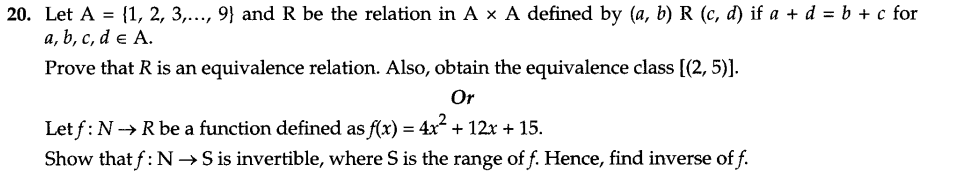21.Compute, using integration, the area bounded by the lines x + 2y = 2, y – x = 1 and 2x + y = 7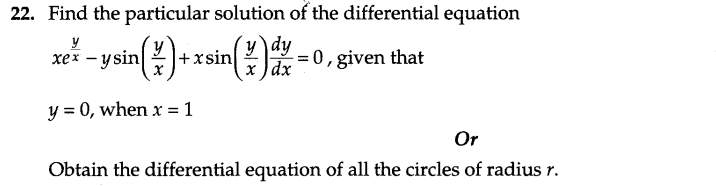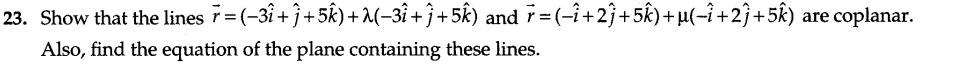24.40% students of a college reside in hostel and the remaining reside outside. At the end of year, 50% of the hostellers got A grade while from outside students, only 30% got A grade in the examination. At the end of the year, a student of the college was chosen at random and was found to get A grade. What is the probability that the selected student was a hosteller ? What value is shown here ?

25.A merchant plans to sell two types of personal computer : a desktop model and a portable model that will cost Rs 25,000 and Rs 40,000 respectively. He estimates that the total monthly demand of computers will not exceed 250 units. Determine the number of units of each type of computers which the merchant should stock to get a maximum profit, if the does not want to invest more than 70 lakh and if his profit on the desktop model is Rs 4,500 and that on portable is Rs 5,000.

26.A jet of enemy is flying along the curve y = x2 + 2 and a soldier is placed at the point (3, 2). Find the minimum distance between the soldier and the jet.

SECTION-A

Question numbers lto6 carry 1 mark each.Ans.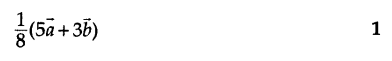Ans. 5 sq.units

3.If P(2, 3, 4) is the foot the of perpendicular from the origin to a plane, then write the vector equation of this plane.
Ans.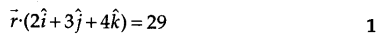Ans.-14Ans.m+n=4

6.Write the differential equation representing the curve y2 = 4ax, where a is an arbitrary constant.
Ans.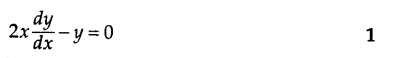SECTION – B

Question numbers 7 to 19 carry 4 marks each.
7.To raise money for an orphanage, students of three schools A, B and C organized an exhibition in their locality, where they sold paper bags, scrap-books and pastel sheets made by them using recycled paper, at the rate of Rs 20, Rs 15 and Rs 5 per unit respectively. School A sold 25 paper-bags, 12 scrap-books and 34 pastel sheets. School B sold 22 paper-bags, 15 scrapbooks and 28 pastel-sheets while school C sold 26 paper-bags, 18 scrap-books and 36 pastel sheets. Using matrices, find the total amount raised by each school.By such exhibition, which values are inculcated in the students ?
Ans.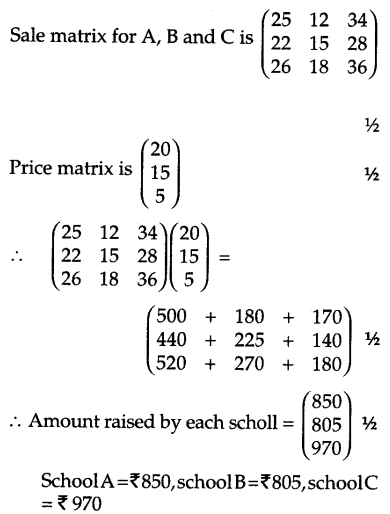Values:i) Helping the Orphans ii)Use of recycled paperAns.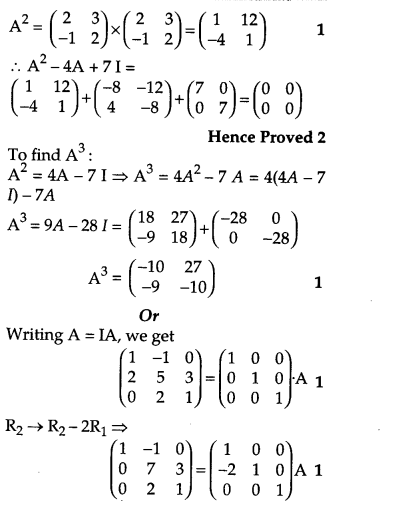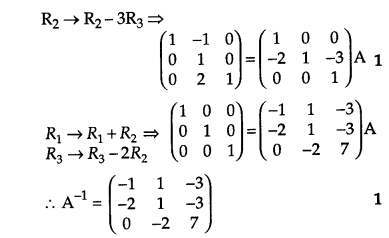Ans.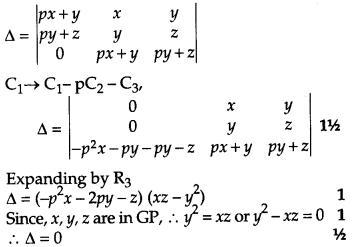Ans.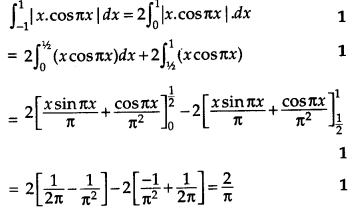Ans.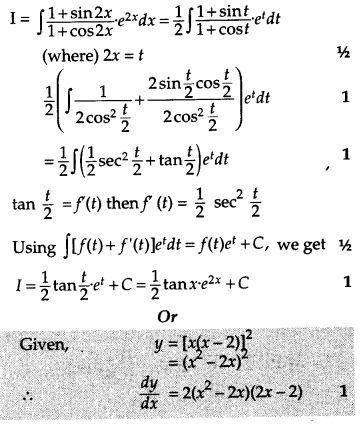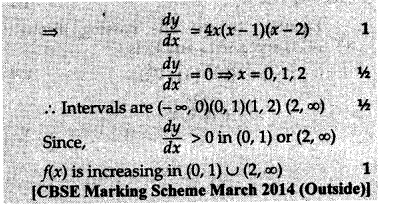12.Consider the experiment of tossing a coin. If the coin shows tail, toss it again but if it shows head, then throw a die. Find the conditional probability of the event that ‘the die shows a number greater than 3’ given that ‘there is at least one head’.
Or
How many times must a man toss a fair coin so that the probability of having at least one head is more than 90% ?

Ans.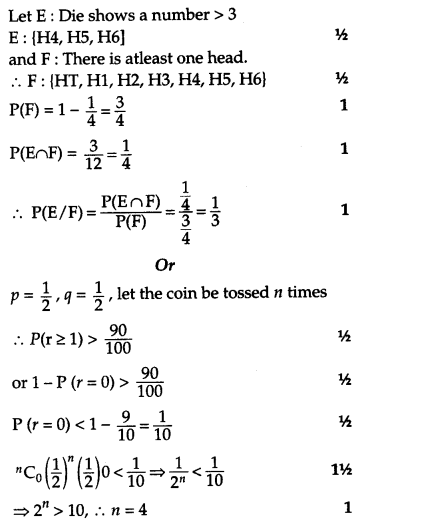Ans.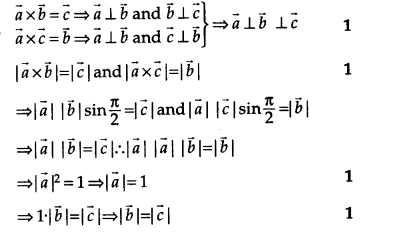Ans.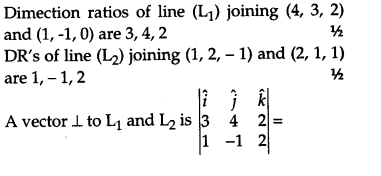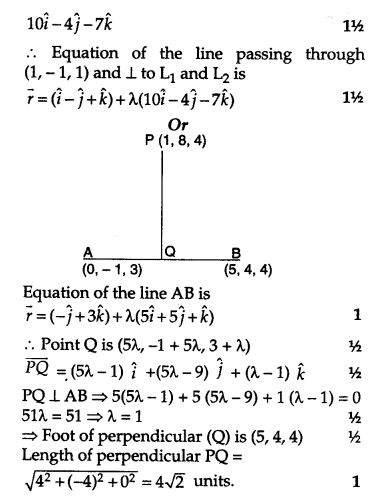Ans.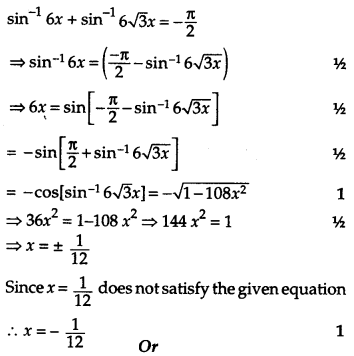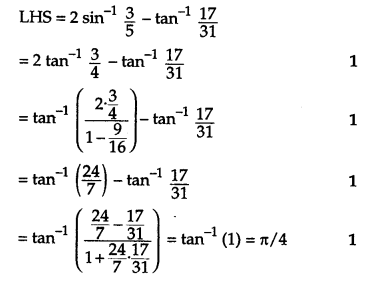16.If x = sin t, y = sin kt, show that,Ans.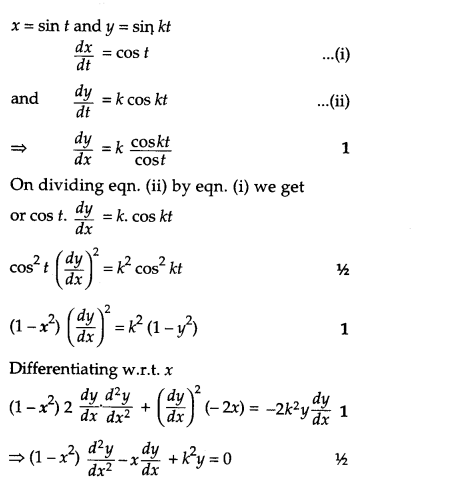Ans.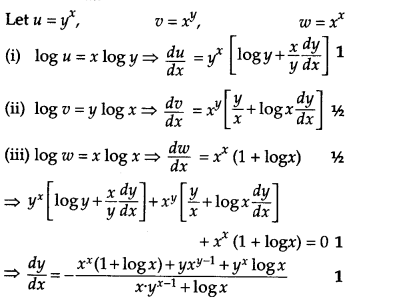Ans.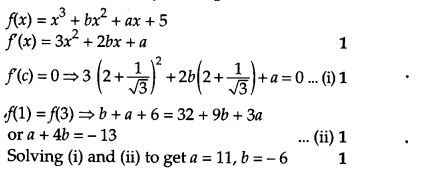Ans.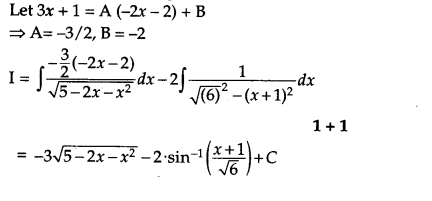SECTION – C

Question numbers 20 to 26 carry 6 marks each.Ans.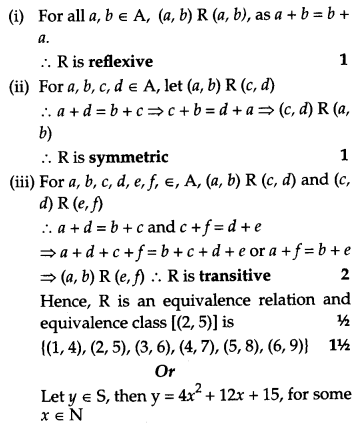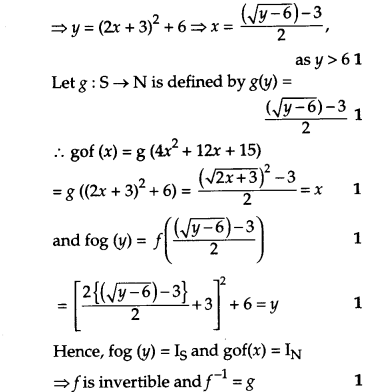21.Compute, using integration, the area bounded by the lines x + 2y = 2, y – x = 1 and 2x + y = 7
Ans.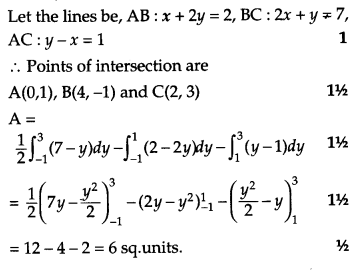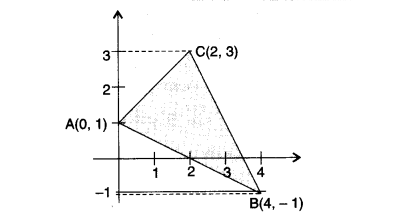Ans.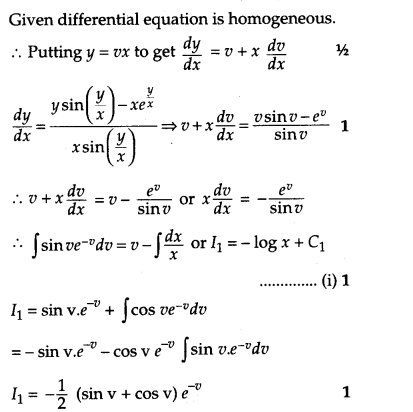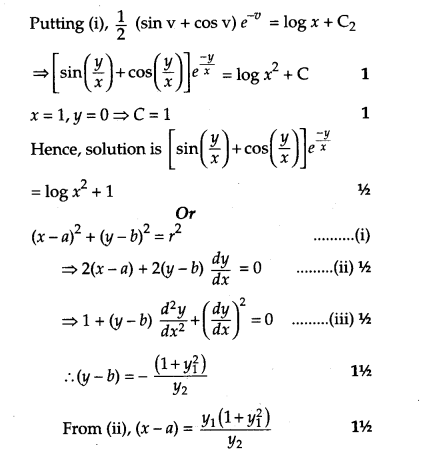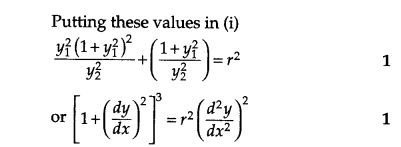Ans.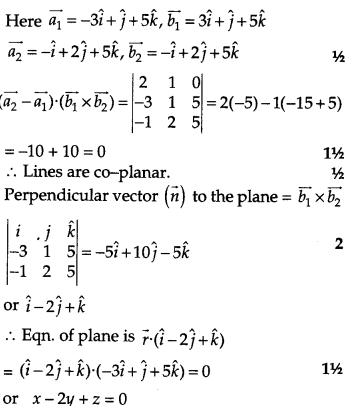24.40% students of a college reside in hostel and the remaining reside outside. At the end of year, 50% of the hostellers got A grade while from outside students, only 30% got A grade in the examination. At the end of the year, a student of the college was chosen at random and was found to get A grade. What is the probability that the selected student was a hosteller ? What value is shown here ?
Ans.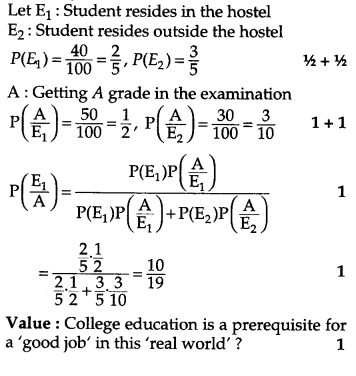25.A merchant plans to sell two types of personal computer : a desktop model and a portable model that will cost Rs 25,000 and Rs 40,000 respectively. He estimates that the total monthly demand of computers will not exceed 250 units. Determine the number of units of each type of computers which the merchant should stock to get a maximum profit, if the does not want to invest more than 70 lakh and if his profit on the desktop model is Rs 4,500 and that on portable is Rs 5,000.
Ans.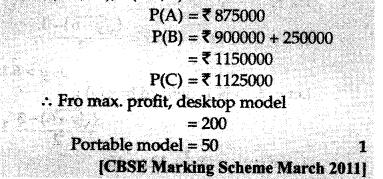26.A jet of enemy is flying along the curve y = x2 + 2 and a soldier is placed at the point (3, 2). Find the minimum distance between the soldier and the jet.
Ans.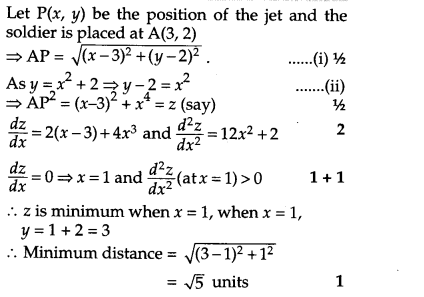Previous articleCBSE Sample Papers for Class 12 Maths 2016 Set 1
Next articleClass 12 Maths CBSE Sample Papers